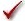## Please enable JavaScript to view this site.

 Navigation: PRINCIPLES OF STATISTICS > Analysis checklists Analysis checklist: Kruskal-Wallis test

The Kruskal-Wallis test is a nonparametric test that compares three or more unpaired or unmatched groups.Read elsewhere to learn about choosing a test, and interpreting the results.

###Are the “errors” independent?

The term “error” refers to the difference between each value and the group median. The results of a Kruskal-Wallis test only make sense when the scatter is random – that whatever factor caused a value to be too high or too low affects only that one value. Prism cannot test this assumption. You must think about the experimental design. For example, the errors are not independent if you have nine values in each of three groups, but these were obtained from two animals in each group (in triplicate). In this case, some factor may cause all three values from one animal to be high or low.

###Are the data unpaired?

If the data are paired or matched, then you should consider choosing the Friedman test instead. If the pairing is effective in controlling for experimental variability, the Friedman test will be more powerful than the Kruskal-Wallis test.

###Are the data sampled from non-Gaussian populations?

By selecting a nonparametric test, you have avoided assuming that the data were sampled from Gaussian distributions, but there are drawbacks to using a nonparametric test. If the populations really are Gaussian, the nonparametric tests have less power (are less likely to detect a true difference), especially with small sample sizes. Furthermore, Prism (along with most other programs) does not calculate confidence intervals when calculating nonparametric tests. If the distribution is clearly not bell-shaped, consider transforming the values (perhaps to logs or reciprocals) to create a Gaussian distribution and then using ANOVA.

###Do you really want to compare medians?

The Kruskal-Wallis test compares the medians of three or more groups. It is possible to have a tiny P value – clear evidence that the population medians are different – even if the distributions overlap considerably.

###Are the shapes of the distributions identical?

The Kruskal-Wallis test does not assume that the populations follow Gaussian distributions. But it does assume that the shapes of the distributions are identical. The medians may differ – that is what you are testing for – but the test assumes that the shapes of the distributions are identical. If two groups have very different distributions, consider transforming the data to make the distributions more similar.